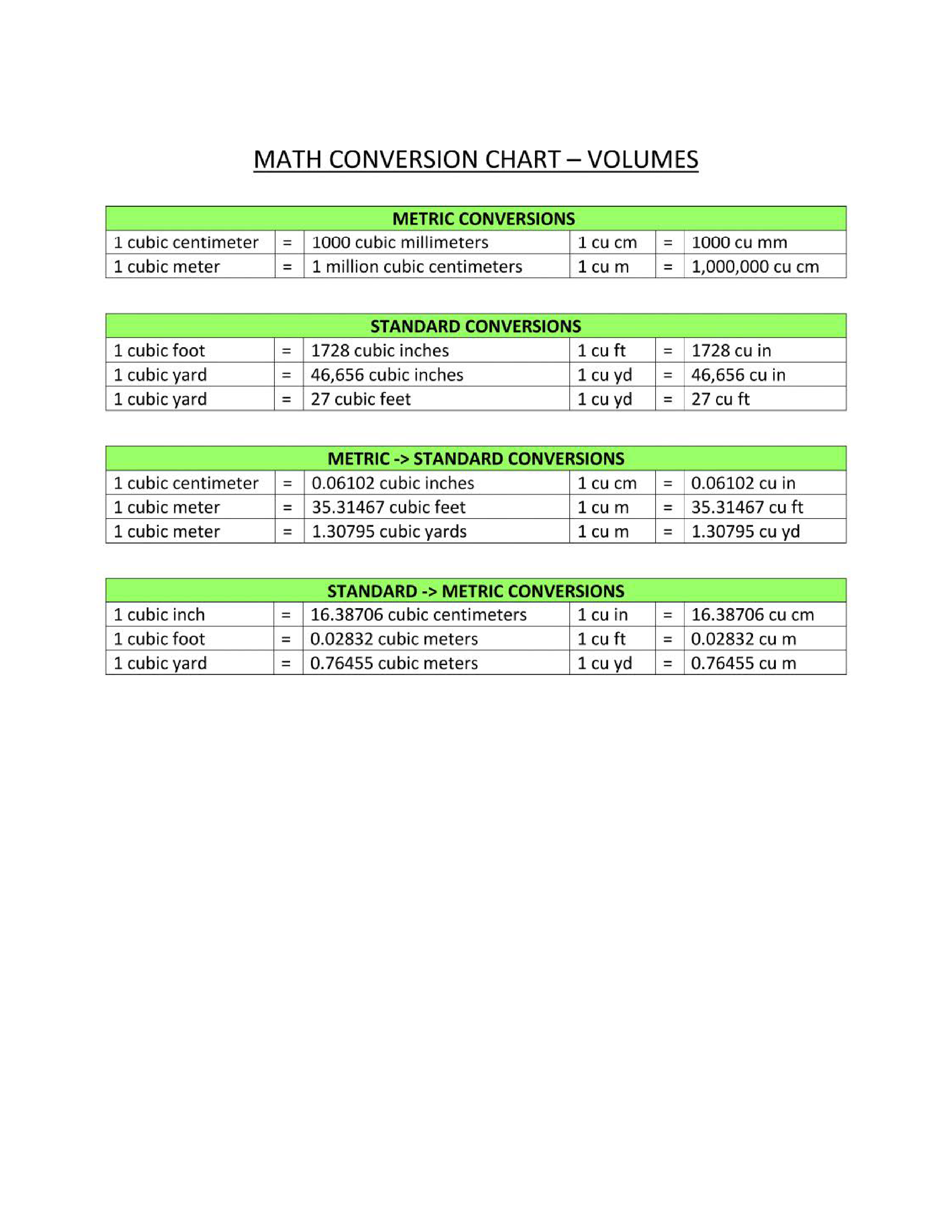# Volume Conversion ChartKlicken Sie auf das Bild zum Vergrößern / Klicken Sie auf die Schaltfläche unten, um mehr Bilder zu sehen

## Speichern, ausfüllen, drucken, fertig!How to convert volume into different metrics? Download this volume conversion reference chart template that will perfectly suit your needs!

Verfügbare Gratis-Dateiformate:

.pdf

• Dieses Dokument wurde von einem Professional zertifiziert
• 100% anpassbar

Benutzer-Bewertung: 8

Malware- und virenfrei. Gescannt von:Life Privat Education Bildung math Mathematik mathematics Mathematik Chemistry Chemie Conversion Chart Umrechnungstabelle metric conversion metrische Umrechnung volume conversion chart Volumenumrechnungsdiagramm math conversion chart Matheumwandlungstabelle math conversions chart Matheumwandlungstabelle conversion chart math Umrechnungstabelle math maths conversion table pdf mathematische Umrechnungstabelle pdf mathematical conversions list Liste der mathematischen Konvertierungen mathematical units conversion table pdf mathematische Einheitenumrechnungstabelle pdf math conversion calculator mathematischer Umrechnungsrechner math conversion formulas mathematische Umwandlungsformeln unit conversion chart printable Einheitenumrechnungstabelle zum Ausdrucken basic metric conversions chart Diagramm der grundlegenden Metrikkonvertierungen metric conversion chart math templates for kindergarten math templates for teachers math templates free download math themed template math worksheet mathematics certificates mathematics formulas math templates maths templates free microsoft powerpoint templates for mathematics microsoft word math templates powerpoint math templates unit conversion chart pdf unit conversion calculator conversion of units of measurement unit conversion chart physics unit conversion chart chemistry unit conversion chart conversion calculator unit converter unit conversion chart download physics all unit conversion chart unit conversion chart sample unit conversion chart template physics unit conversion chart

How to convert volume into different metrics? Download this volume conversion reference chart template that will perfectly suit your needs!

Often, conversion is needed between units used in the metric system and the imperial system. Therefore, are common online calculators or offline metric conversion charts available. This Metric conversion chart aims to help to convert units of measurement with Metric Converter and Metric Conversion Table into Imperial system units and to better understand alternative measurements that they are unfamiliar with.

For example for Volume Conversion Chart, shows:
• metric volume conversions;
• imperial volume conversions;
• standard volume conversions;
• metric to standard conversions;
• standard to metric conversions.

This Volume Conversion Chart is intuitive, ready-to-use, and structured in a smart way. Try it now and let this mathematics template inspire you. We certainly encourage you to use this Volume Conversion Chart for your own benefit and are confident it will fit your needs

Download this Volume Conversion Chart now and print and place it in sight!

HAFTUNGSAUSSCHLUSS
Nichts auf dieser Website gilt als Rechtsberatung und kein Mandatsverhältnis wird hergestellt.

Wenn Sie Fragen oder Anmerkungen haben, können Sie sie gerne unten veröffentlichen.mehr Themen